# Load Balancing as an Optimization Problem: GSO Solution

METHODOLOGY1. INTRODUCTION

In this chapter, we presented a novel methodology which considers load balancing as an optimization problem. A stochastic approach, Glowworm swarm optimization (GSO) is employed to solve the above mentioned optimization problem. In the proposed method, excellent features of various existing load balancing algorithms as discussed chapter 2 are also integrated.

1. PROPOSED METHODOLOGY

There are numerous cloud computing categories. This work mainly focuses on a public cloud. A public cloud is based on the typical cloud computing model, and its services provided by service provider . A public cloud will comprises of several nodes and the nodes are in different physical locations. Cloud is partitioned to manage this large cloud. A cloud consists of several cloud partition with each partition having its own load balancer and there is a main controller which manage all these partition.

3.2.1 Job Assignment Strategy

Algorithm for assigning the jobs to cloud partition as shown in Fig. 2

Step 1: jobs arrive at the main controller

Step 2: choosing the cloud partition

Step 3: if cloud partition state is idle or normal state then

Step 4: jobs arrive at the cloud partition balancer.

Step 5: assigning the jobs to particular nodes based on the strategy.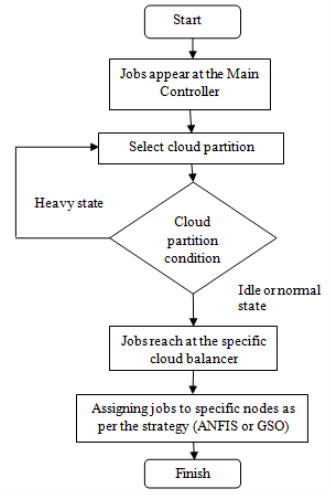Figure 3.1: Flowchart of Proposed Job Assignment Strategy.

In cloud, Load Balancing is a technique to allocate workload over one or more servers, network boundary, hard drives, or other total resources. Representative datacenter implementations depends on massive, significant computing hardware and network communications, which are subject to the common risks linked with any physical device, including hardware failure, power interruptions and resource limits in case of high demand.

High-quality of load balance will increase the performance of the entire cloud.Though, there is no general procedure that can work in all possible different conditions. There are several method have been employed to solve existing problem.

Each specific method has its merit in a specific area but not in all circumstances. Hence, proposed model combines various methods and interchanges between appropriate load balance methods as per system status. Here, the idle status uses an Fuzzy Logic while the normal status uses a global swarm optimization based load balancing strategy.

1. Load Balancing using Fuzzy Logic

When the status of cloud partition is idle, several computing resources are free and comparatively few jobs are receiving. In these circumstances, this cloud partition has the capability to process jobs as fast as possible so an effortless load balancing method can be used.

The fuzzification process is carried out by fuzzifier that transforms two types of input data like assigned load and processor speed of Virtual Machine (VM) and one output as balanced load which are required in the inference system shown in figure 3.2, figure 3.3 and figure 3.4 respectively. By evaluating the load and processor speed in virtual machine in our proposed work like two input parameters to produce the better value to equalize the load in cloud environment, fuzzy logic is used. These parameters are taken for inputs to the fuzzifier, which are needed to estimate the balanced load as output as shown in figure 3.4.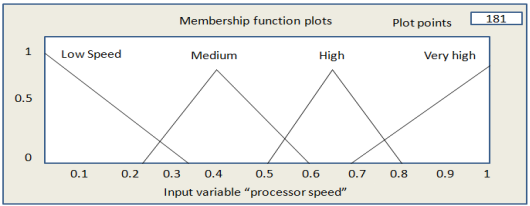Figure 3.2: Membership input function of Processor Speed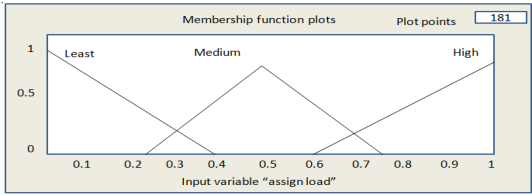Figure 3.3: Membership input function of Assigned Load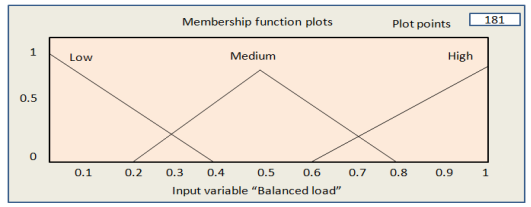Figure 3.3: Membership output function of Balanced Load

To affiliate the outputs of the inferential rules  , low-high inference method is employed. A number of IF-THEN rules are determined by making use of the rule-based fuzzy logic to get the output response with given input conditions, here the rule is comprised from a set of semantic control rules and the supporting control objectives in the system.

1. If (processor_speed is low) and (assigned_load is least) then (balanced_load is medium)
2. If (processor_speed is low) and (assigned_load is medium) then (balanced_load is low)
3. If (processor_speed is low) and (assigned_load is high) then (balanced_load is low)
4. If (processor_speed is Medium) and (assigned_load is least) then (balanced_load is high)
5. If (processor_speed is Medium) and (assigned_load is medium) then (balanced_load is medium)
6. If (processor_speed is Medium) and (assigned_load is high) then (balanced_load is low)
7. If (processor_speed is high) and (assigned_load is least) then (balanced_load is high)
8. If (processor_speed is high) and (assigned_load is medium) then (balanced_load is medium)
9. If (processor_speed is high) and (assigned_load is high) then (balanced_load is medium)
10. If (processor_speed is very_high) and (assigned_load is least) then (balanced_load is high)
11. If (processor_speed is very_high) and (assigned_load is medium) then (balanced_load is high)
12. If (processor_speed is very_high) and (assigned_load is high) then (balanced_load is medium)

As shown above, there are 12 potential logical output response conclusions in our proposed work. The Defuzzification is the method of changing fuzzy output set into a single value and the smallest of minimum (SOM) procedure is employed for the defuzzification.

The total sum of a fuzzy set comprises a range of output values that are defuzzified in order to decode a single output value. Defuzzifier embraces the accumulated semantic values from the latent fuzzy control action and produces a non-fuzzy control output, which enacts the balanced load associated to load conditions.

The defuzzification process is used to evaluate the membership function for the accumulated output. The algorithm-1 is defined to manage the load in Virtual machine of cloud computing as follows:

Begin

Request_to_resource()

L1

If (resource free)

Begin

Estimate connection_string()

Select fuzzy_rulebase()

Return resource

End

Else

Begin

If (Anymore resource found)

Select_next_resource()

Go to L1

Else

Exit

End

End

The proposed algorithm starts with request a connection to resource. It tests for availability of resource. It Calculate the connection strength if the resource found. Then select the connection, which is used to access the resource as per processor speed and load in virtual machine using fuzzy logic.

1. Load Balancing using GSO (Glowworm Swarm Optimization)

When the status of cloud partition is normal, tasks arrives with faster rate compare to idle state and the condition becomes more complex, thus a novel strategy is deployed for load balancing. Each user desired his job in the shortest time; as a result the public cloud requires a strategy that can finish the job of all users with adequate response.

In this optimization algorithm, each glowworm i is distributed in the objective function definition space . These glowworms transfer own luciferin values and have the respective scope called local-decision range . As the glow searches in the local-decision range for the neighbor set, in the neighbor set, glow attracted to the neighbor with brightest glow. That is glow selects neighbor whose luciferin value greater than its own, and the flight direction will change each time different will change with change in selected neighbor.

Each glowworm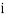encodes the object function value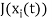at its current location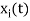into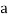luciferin value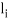and advertises the same within its neighborhood. The neighbor’s set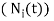of glowwormcomprises of those glowworms that have comparatively a higher luciferin value and that are situated within a dynamic decision range and their movements are updated by equation (8) at each iteration.

Local-decision range update: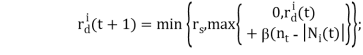(8)

and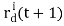is the glowworm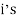local-decision range at the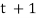iteration,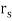is the sensor range,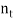is the neighbourhood threshold, the parameter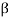generates the rate of change of the neighborhood range. Local-decision range consist of the following number of glow: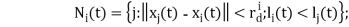(9)

and,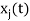is the glowwormposition at the t iteration,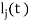is the glowwormluciferin at the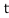iteration.; the set of neighbours of glowwormcomprises of those glowworms that have a comparatively higher luciferin value and that are situated within a dynamic decision range whose range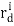is defined above by a circular sensor range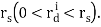Each glowworm as given in equation (10), i elects a neighbor j with a probability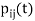and process toward it as:

Probability distribution used to select a neighbor: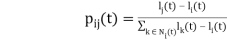(10)

Movement update: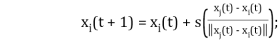(11)

Luciferin-update: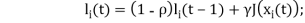(12)

and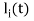is a luciferin value of glowwormat eachiteration,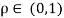leads to the reflection of the accumulative goodness of the path . This path is followed by the glowworms in their ongoing luciferin values, the parameter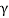only ascends the function fitness values,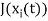is the value of test function.

In this optimization algorithm, each glowworm is distributed in the objective function definition space . These glowworms transfer own luciferin values and have the respective scope called local-decision range . As the glow searches in the local-decision range for the neighbor set, in the neighbor set, glow attracted to the neighbor with brightest glow. That is glow selects neighbor whose luciferin value greater than its own, and the flight direction will change each time different will change with change in selected neighbor. Figure 3.4 shows the flowchart of GSO algorithm.

In the context of load balancing for cloud computing GSO algorithm check the status of the server simultaneously if it is free. For example a user wants to download a file size of 50 MB. It checks by iteration if user gets entered in server, it gets the message as achieve target.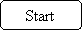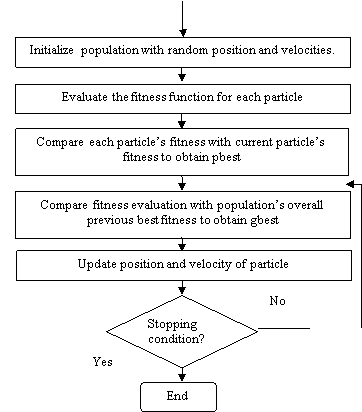Figure 3.4: Flowchart of GSO

The post Load Balancing as an Optimization Problem: GSO Solution appeared first on mynursinghomeworks.